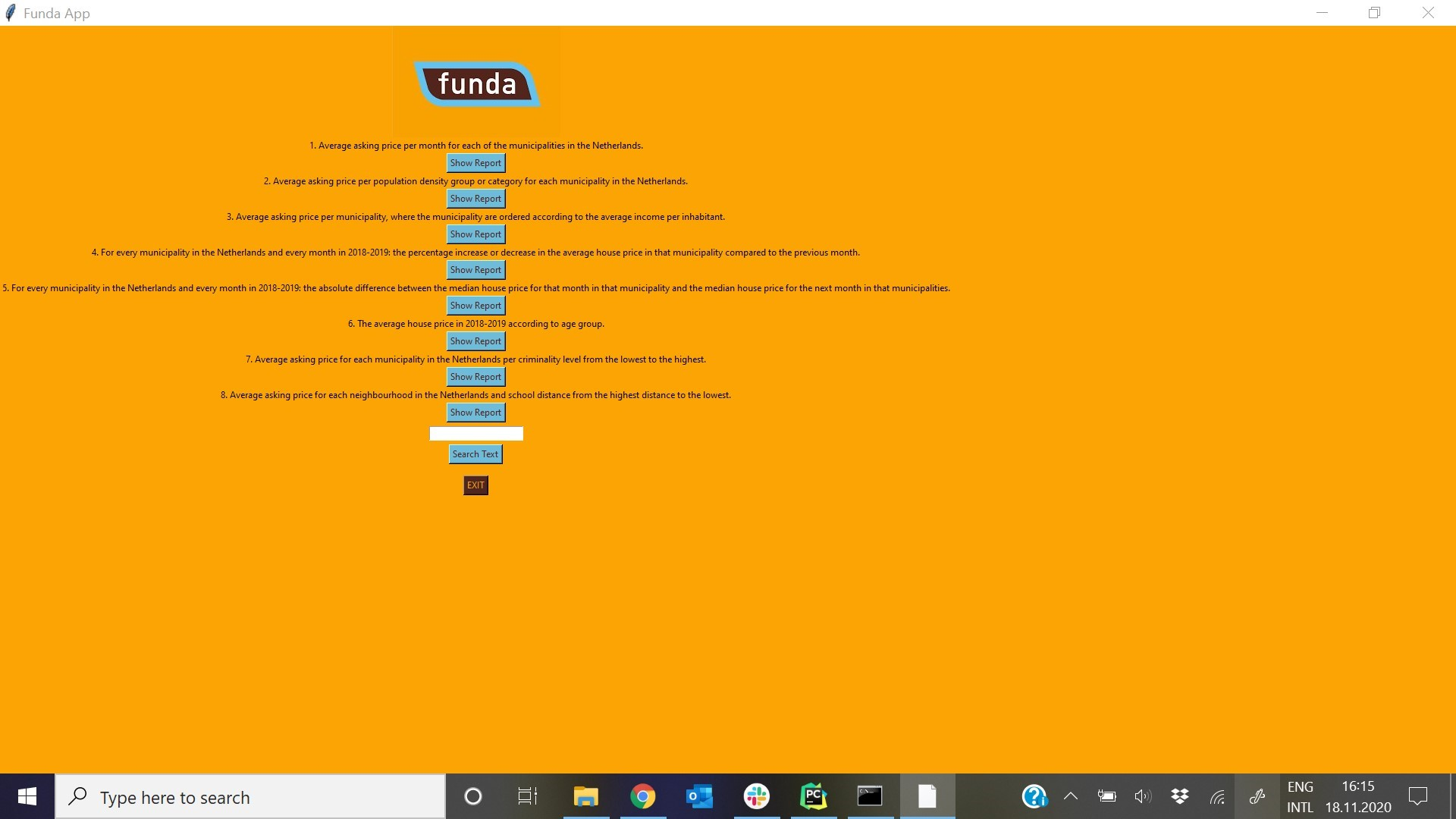# How prevent window size change after plot function?I am making reporting application with tkinter python and postgres. Queries built in postgres and executed through python. Also developed a plot function, which takes to variables a displays them in a plot. The problem is that if the plot function is called the tkinter window widgets (label, entry, button) diminish in size. This is the plot function. Tried to change the figsize but nothing changed. How can I fix it?

``````def plot(x, y, plot_name):
fig = plt.figure(figsize=(10, 10))
plt.scatter(x, y,
linewidths=1, alpha=.7,
edgecolor='k',
s=200)
plt.xlabel(x.name, fontsize=16)
plt.ylabel(y.name, fontsize=16)
fig.savefig(plot_name + '.jpg')
return plt.show()

window = Tk()

window.title("Funda App")

w, h = window.winfo_screenwidth(), window.winfo_screenheight()
window.geometry("%dx%d+0+0" % (w, h))

window['bg'] = '#fba404'
``````

EDIT This is how the plot function is called and the below function is called by clicking at a button.

``````def avg_price_per_gemeente_ordered_average_income_per_inhabitant(cur):
query = """
select avg(koopprijs) as average_price, gemeente.gemeentenaam as municipality, avg(income) as average_income
from funda inner join postcode on funda.postcode = postcode.postcode
inner join gemeente on postcode.gemeente = gemeente.gemcode inner join cbs_income_by_gemeente on
cbs_income_by_gemeente.gemeente = gemeente.gemcode
group by income, gemeente.gemeentenaam order by avg(income) DESC;
"""

cur.execute(query)
data = pd.DataFrame(cur.fetchall(),
columns=["average_price", "municipality", "average_income"])
data.to_csv("3.avg_price_per_gemeente_ordered_average_income_per_inhabitant.csv", index=False)
plot(data['average_price'], data['average_income'], 'avg_price_per_gemeente_ordered_average_income_per_inhabitant')
response = "Check the results in 3.avg_price_per_gemeente_ordered_average_income_per_inhabitant.csv"
return messagebox.showinfo("Information", response)

``````

EDIT 2

in here is the code of the button clicked.

``````lbl = Label(window, text="3. Average asking price per municipality, where the municipality are ordered according to the average income per inhabitant.", bg='#fba404')
lbl.grid(column=0, row=5)
btn = Button(window, text="Show Report", command= lambda: avg_price_per_gemeente_ordered_average_income_per_inhabitant(cur),bg='#6fbbda', fg='#4f251f')
btn.grid(column=0, row=6)
``````